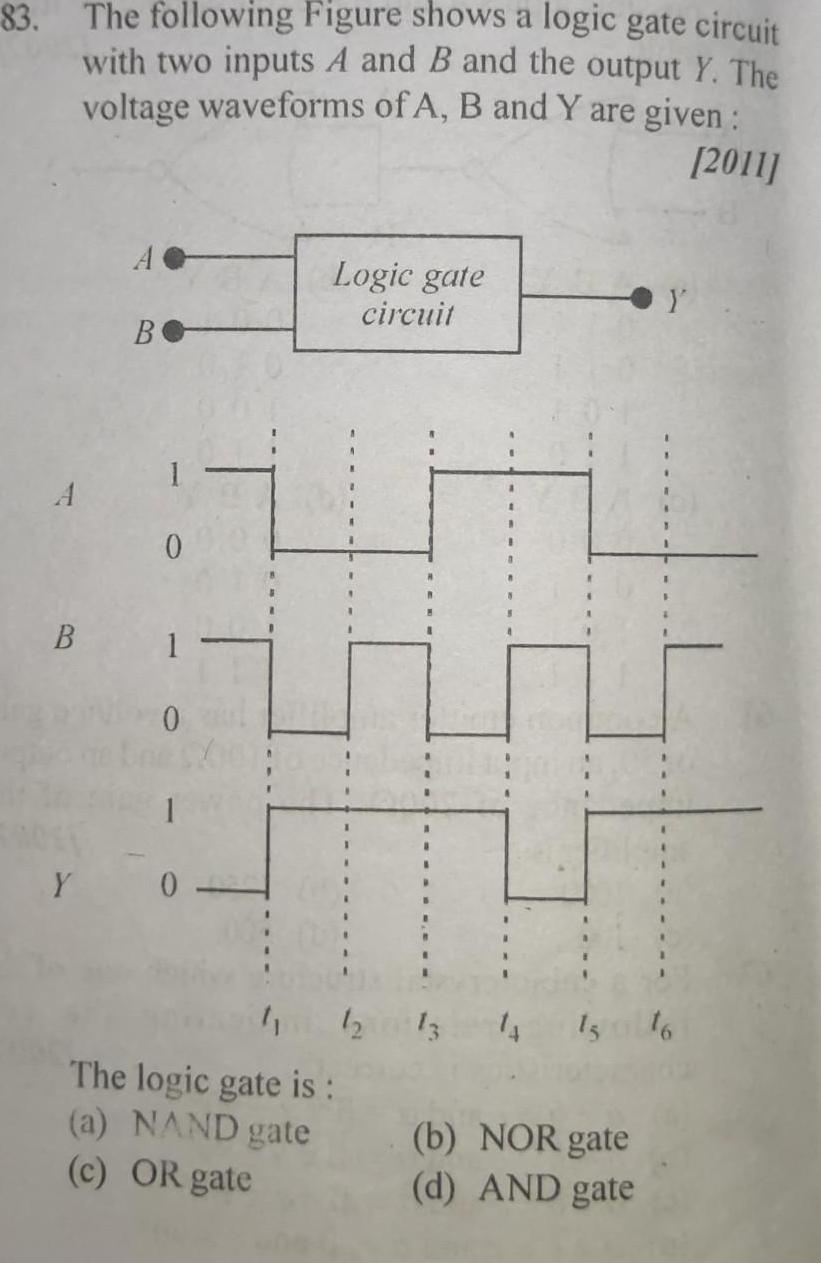Question:

# 83 The following Figure shows a logic gate circuit with two

Last updated: 6/8/202383 The following Figure shows a logic gate circuit with two inputs A and B and the output Y The voltage waveforms of A B and Y are given 2011 A B A B 0 1 1 Y 0 Logic gate circuit The logic gate is a NAND gate c OR gate 13 14 b NOR gate d AND gate